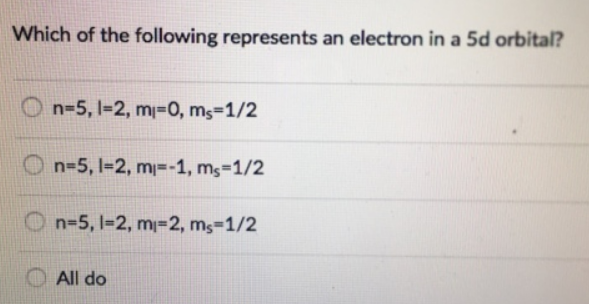# Problem: Which of the following represents an electron in a 5d orbital? (a) n=5, I=2, ml=0, ms=1/2 (b) n=5, I=2, ml=-1, ms=1/2 (c) n=5, I=2, ml=2, ms=1/2 (d) All do

🤓 Based on our data, we think this question is relevant for Professor All Professors' class at HCC.

###### FREE Expert Solution###### Problem Details

Which of the following represents an electron in a 5d orbital?

(a) n=5, I=2, ml=0, ms=1/2

(b) n=5, I=2, ml=-1, ms=1/2

(c) n=5, I=2, ml=2, ms=1/2

(d) All do